# Objective of this tutorial

In this tutorial we want to show how to combine different formulas/variations and how to change parameters of formulas. Please note, that there is no difference between formulas and variations, but I prefer the term "formula" because it is more precise.

# Create a new empty fractal with a blurring formula

To get started, create a new empty fractal by hitting the New from scratch-button. Have a look at the Gradient-tab, when you do not like the randomly created gradient, just hit the New from scratch again. And again if you are not satisfied yet...

Add a new transform by pressing the Add-button on the Transformations-tab. Change the variation 1 from "linear3D" to "circleblur", you should see a filled circle in the viewport.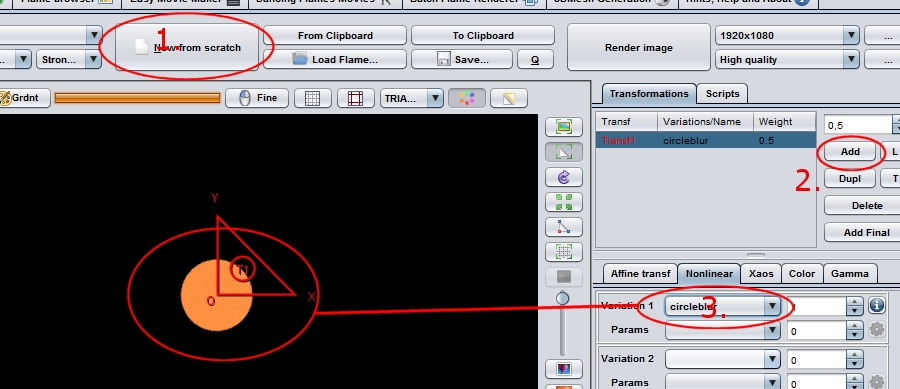But, when you drag the controls around, you will see, that the shape does not change. But, why? To understand this, we must look at formulas more close. There are different types.

## Types of formulas/variations

There are two major categories of formulas:

1. transforming formulas: formulas which change an existing part of the fractal, where the detail of the original is not changed.

For example, a wave-transformation applied to an existing fractal will just make it "wavy", but not increase or decrease the detail very much. I.e., a transforming formula contributes to the overall transform.

1. blurring formulas: formulas who completely ignore the existing fractal and create new shape.

For example, "circleblur" will always create a filled circle, and not transform the existing structure, it will replace it. I.e., used in this simple scenario, a blurring formula overwrites the transforms, so that it is not a conventional transform anymore.

That also means that it isn't possible to create fractals by using only blurring formulas, because you need transforms to create the typical fractal (i. e. self-similar) structure. You need some transform, which transforms some transformed stuff, which was transformed by ..., and so on. But blurring formulas, when used at the right place, are really great for constructing flames from scratch.

# Add a second transform with a transforming formula

Now add a second transform and change the variation 2 from "linear3D" to "julian", you should not see much difference in the viewport yet.

## Formulas with parameter

The formula you chose is a formula with additional parameters. For such formulas you use the listbox below the listbox which show the name the of the formula to select a a parameter, and the edit-field right of it to change the value of this parameter.
Please note, that not all parameters are just numbers, some formulas even have complex structures like images as parameters, in this case you may find more controls right of this field.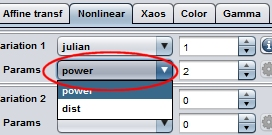Please change the value of the parameter power of the "julian"-formula to 2. Then use your mouse to modify the seconds transform (the one with "julian"-formula on it). Enlarge the control, rotate it and move it in the viewport until you get something like this: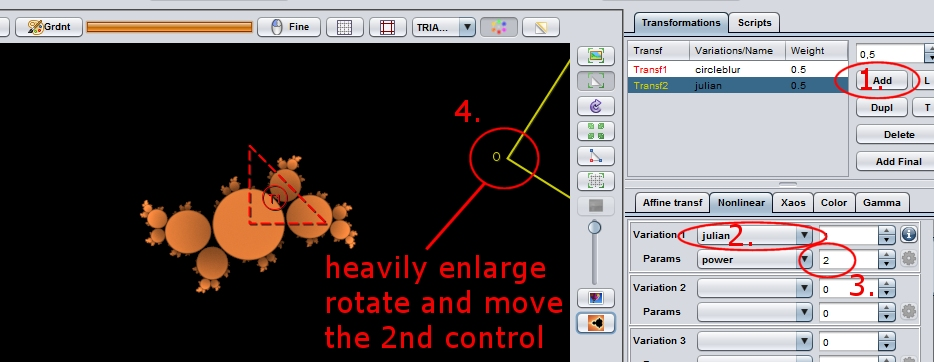The fractal is still monochrome, to change this enter the Color-tab on the Transformations-tab, and modify the Color-slider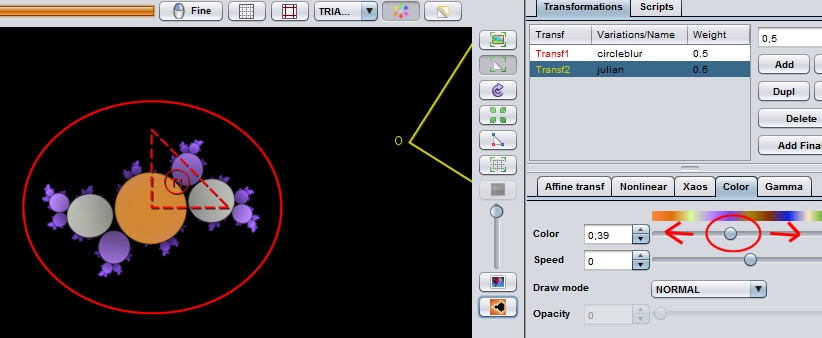## Changing formula params

Now let's play again with the parameters of the "julian"-variation to show how small parameter-changes can cause huge differences in the final result. Change "power" to 3, and "dist" to 1.76.

You have three choices for how to do this:

1. Enter the value directly (which is only a choice if you know the value, i. e. not for playing around)
2. Enter the field with the mouse, click and hold the left mouse-button and move the mouse to decrease/increase the value (best for playing around)
3. Use the small arrow-buttons to slightly decrease/increase the value (best for fine-adjustments)

Your flame should look like this (if not you may compare your result with the flame-params which are supplied in the appendix):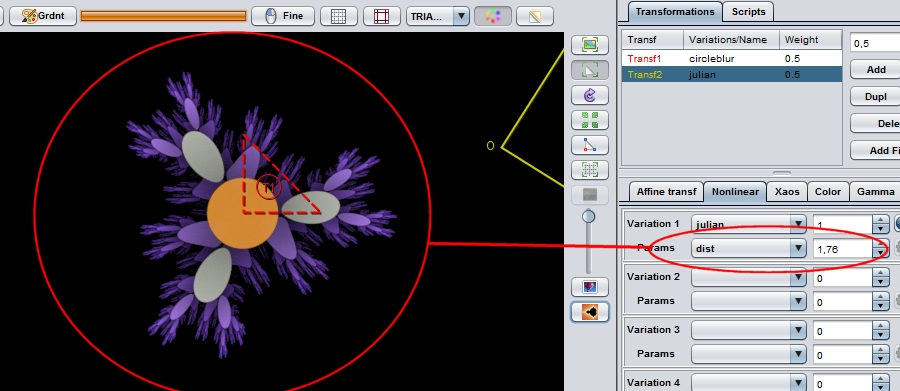### Fine adjustment of parameters

Sometimes the parameter-changes done by using the mouse or the arrow-buttons are too large for, even if done carefully. For this purpose you can activate the Fine-button at the top of the editor-area. All changes, you do now, are scaled down by a factor of approximately 10.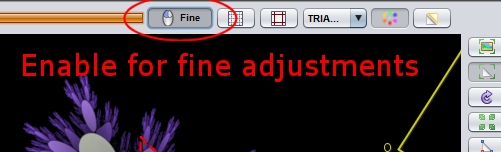# Conclusion

We have learned how to use different formulas in one fractal, how to change formulas and that there two types of formula (transforming and blurring formula). And we saw how to change parameters of formulas and that also small changes to parameters of formulas may have a large impact on the final image.

# Appendix

## Params of the final sample flame

```<flame smooth_gradient="0" version="JWildfire 2.34 (22.02.2015)" size="643 362" center="0.0 0.0" scale="35.17734553722945" rotate="0.0" filter="0.0" filter_kernel="GAUSSIAN" quality="10.0" background="0.0 0.0 0.0" bg_transparency="0" brightness="4.0" saturation="1.0" gamma="4.0" gamma_threshold="0.01" vibrancy="1.0" contrast="1.0" white_level="220.0" temporal_samples="1.0" cam_zoom="1.0" cam_pitch="0.0" cam_yaw="0.0" cam_persp="0.0" cam_xfocus="0.0" cam_yfocus="0.0" cam_zfocus="0.0" cam_pos_x="0.0" cam_pos_y="0.0" cam_pos_z="0.0" cam_zpos="0.0" cam_dof="0.0" cam_dof_area="0.5" cam_dof_exponent="2.0" cam_dof_shape="BUBBLE" cam_dof_scale="1.0" cam_dof_rotate="0.0" cam_dof_fade="1.0" shading_shading="FLAT" antialias_amount="0.75" antialias_radius="0.36" post_symmetry_type="NONE" post_symmetry_order="3" post_symmetry_centre_x="0.0" post_symmetry_centre_y="0.0" post_symmetry_distance="1.25" post_symmetry_rotation="6.0" frame="1" frame_count="300" mixer_mode="OFF" >
<xform weight="0.5" color="0.0" mod_gamma="0.0" mod_gamma_speed="0.0" mod_contrast="0.0" mod_contrast_speed="0.0" mod_saturation="0.0" mod_saturation_speed="0.0" symmetry="0.0" circleblur="1.0" coefs="1.0 0.0 0.0 1.0 0.0 0.0" chaos="1.0 1.0" />
<xform weight="0.5" color="0.39" mod_gamma="0.0" mod_gamma_speed="0.0" mod_contrast="0.0" mod_contrast_speed="0.0" mod_saturation="0.0" mod_saturation_speed="0.0" symmetry="0.0" julian="1.0" julian_power="3" julian_dist="1.76" coefs="1.8747239284667137 1.1869473754012025 -1.1869473754012025 1.8747239284667137 3.3237513474667604 -0.8341460910630871" chaos="1.0 1.0" />
<palette count="256" format="RGB" >
F7883EF6873CF6863AF58538F48436F38334F38232F28130F1802DF07F2BF07D29EF7C27
EE7B25ED7A23ED7921EC781FEB771DEA761BEA7519E97417E87315E77213E77110E6700E
E56F0CE46E0AE46F0BE37511E27A16E1801CE18521E08B27DF912CDF9632DE9C37DDA13D
DCA742DCAC48DBB24DDAB753D9BD58D9C25ED8C863D7CE69D6D36ED6D974D5DE79D4E47F
C1A2E8C09EECBF9AF0BE96F4BE91F8BD8DFBBA8AFBB887FBB584FBB381FBB17EFAAE7BFA
AC78FAA975FAA772FAA56FFAA26BFAA068FA9E65F99B62F9995FF9965CF99459F99256F9
8F53F98D50F98A4DF98849F88646F88343F88140F88141F28243E98446E08548D6874BCD
894DC38A50BA8C53B08D55A78F589E905A94925D8B935F8195627896656F986765996A5C
9B6C529C6F499E723F9F7436A1772DA27923A47C1AA57E10A78107A67E07A47B07A37807
A27507A072079F6E079E6B069C68069B65069A6206985F06975C06965906945606935306
925006904D068F49068E46068C43058B40058A3D05883A058737058634058332097E3112
79311B753024702F2D6B2F36662E3F612E485C2D51572C5A532C634E2B6C492B75442A7E
3F2A873A29903628993128A22C27AB2727B42226BD1D25C61925CF1424D80F24E10A23EA
1129EB1A31E92439E82D40E63748E54050E34A57E2535FE05D67DF666EDD7076DC797EDA
8386D98D8DD89695D6A09DD5A9A4D3B3ACD2BCB4D0C6BBCFCFC3CDD9CBCCE2D2CAECDAC9